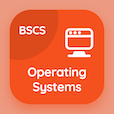Computer Science Courses Online

Database Management System Practice Tests

Database Management System Online Tests

The Book Hashing Techniques Multiple Choice Questions (MCQ Quiz) with answers, Hashing Techniques MCQ Quiz PDF download to study online database management system degree courses. Practice Disk Storage, File Structures and Hashing Multiple Choice Questions and Answers (MCQs), Hashing Techniques quiz answers PDF for online degrees. The e-Book Hashing Techniques MCQ App Download: hashing techniques, ordered records, disk file records, introduction to disk storage test prep for information and communication technology.

The MCQ: In linear hashing, the formula of file load factor is PDF, "Hashing Techniques" App Download (Free) with l = r⁄ (bfr * n), l = r⁄ (bfr + n), l = r⁄ (bfr - n), and l = r⁄ (bfr * 2n) choices for online degrees. Study hashing techniques quiz questions, download Google eBook (Free Sample) for associates in computer science.

## Database Management System MCQs: Hashing Techniques Quiz Questions

MCQ: In linear hashing, the formula of file load factor is

A) l = r⁄ (bfr * N)
B) l = r⁄ (bfr + N)
C) l = r⁄ (bfr - N)
D) l = r⁄ (bfr * 2N)

MCQ: The hashing technique which allocates fixed number of buckets is classified as

A) dynamic hashing
B) static hashing
C) external hashing
D) internal hashing

MCQ: The hashing technique which allows increase or decrease in number of buckets without a need of directory is classified as

A) global depth hashing
B) linear hashing
C) relative hashing
D) local depth hashing

MCQ: In linear hashing, the formula used to calculate number of records if the blocking factor, loading factor and file buckets are known is as

A) r =l + bfr + N
B) r =l - bfr - N
C) r =l + bfr - N
D) r =l * bfr * N

MCQ: If the global depth is more than local depth then the operation must be performed in directory array is

B) subtracting
C) halving
D) doubling

### Practice Tests: Database Management System Exam Prep

Download Database Management System Quiz App, Operating Systems MCQ App, and Digital Logic Design MCQs App to install for Android & iOS devices. These Apps include complete analytics of real time attempts with interactive assessments. Download Play Store & App Store Apps & Enjoy 100% functionality with subscriptions!